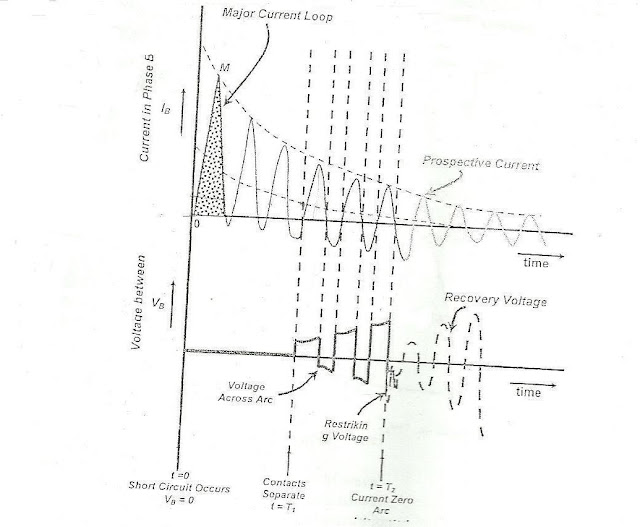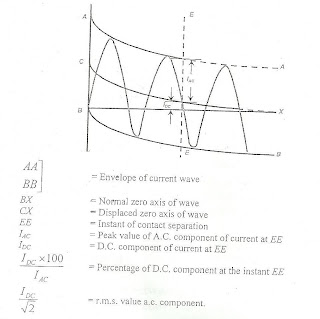### Rated and Short Circuit Current

4. Rated characteristic of circuit breakers (part2)
4.4 Rated Normal Current (Rated Current)
The rated normal current of  a circuit breaker is the r.m.s value of the current which the circuit breaker can carry continuously and with temperature rise of the various parts within specified limits. Preferred values of rated current in Arms are 400, 630, 800, 1250, 1600, 2000, 2500, 3150, and 4000.
The design of contacts and other current carrying parts in the interrupter of the circuit breaker are generally based on the limits of the temperature rise. For a given cross-section of the conductor and a certain value of current, the temperature rise depends upon the conductivity of the material. Hence, high conductivity material is preferred for current carrying parts. The cross-section of the conductors should be increased for materials with lower conductivity. The use of magnetic materials in close circuits should be avoided to prevent heating due to hysteresis loss and eddy currents. The rated current of a circuit breaker is verified by conducting temperature rise tests.
The rated short circuit breaking current of a circuit breaker is the highest rms value of short circuit current which the circuit breaker is capable of breaking under specified conditions of transient recovery voltage and power frequency voltage. It is expressed in KArms at contact separation.

4.5 Rated Short Circuit Breaker Current
Referring to Fig. 2, the short circuit current has a certain value at the instant of contact separation, (t = T1).  The breaking current refers to value of current at the instant of the contact separation.
The transient recovery voltage refers to the transient voltage appearing across  the circuit breaker pole immediately after the arc interruption.Figure 2  Oscillogram of  Current and Voltage during fault clearing

The rated values of transient recovery voltage are specified for various rated voltage of circuit breakers. For specified conditions of rated TRV and rated power frequency recovery voltage, a circuit breaker has a certain limit of breaking current. This limit is determined by conducting short circuit type tests on the circuit breaker. The waveforms of short circuit current are obtained during the breaking test. The evaluation of the breaking current is explained in Fig. 3. The breaking current is expressed by two values :

1.     The r.m.s value of a.c. component at the instant of contact separation EE, given by
2.     The percentage d.c. component at the instant of contact separation given by

The r.m.s values of a.c. components are expressed in KA. the standard values being 8, 10, 12.5, 16, 20, 25, 31.5, 40, 45, 63, 80 and 100KA.
The earlier practice was to express the rated breaking capacity of a circuit breaker in terms of MVA given as follows
MVA = √3 x KV x KA
Where MVA = Breaking capacity of a circuit breaker kV
kV = Rated voltage
kA = Rated breaking currentFigure 3   Dimention of breaking current.

This practice of specifying the breaking capacity in terms of MVA is convenient while calculating the fault levels. However, as per the revised standards, the breaking capacity is expressed in KA for specified conditions of TRV and this method takes into account both breaking current and TRV.
While selecting the circuit breaker for a particular location in the power system the fault level at that location is determined. The rated breaking current can then be selected from standard range.

4.6 Rated Short Circuit Making Current
It may so happen that circuit breaker may close on an existing fault. In such cases the current increase to the maximum value at the peak of first current loop. The circuit breaker should be able to close without hesitation as contact touch. The circuit breaker should be able to withstand the high mechanical forces during such a closure. These capabilities are proved by carrying out making current test. The rated short circuit making current of a circuit breaker is the peak value of first current loop of short circuit current (I pk)Which the circuit breaker is capable of making at its rated voltage.
The rated short circuit making current should be least 2.5 times the r.m.s. value of a.c. component of rated breaking current .
Rated making current  = 1.8 x √2 x Rated short circuit breaking
= 2.5 x Rated short circuit breaking current
In the above equation the factor √2 convert the r.m.s  value to peak value. Factor 1.8 takes into account the doubling effect of short circuit current with consideration to slight drop in current during the first quarter cycle .

Sponsored links :

#### 1 comment:

1.Great Blog,Thanks for sharing such beautiful information with us. I hope to will share some more information about Arc flash temperature. Please visit our website Arc flash temperature